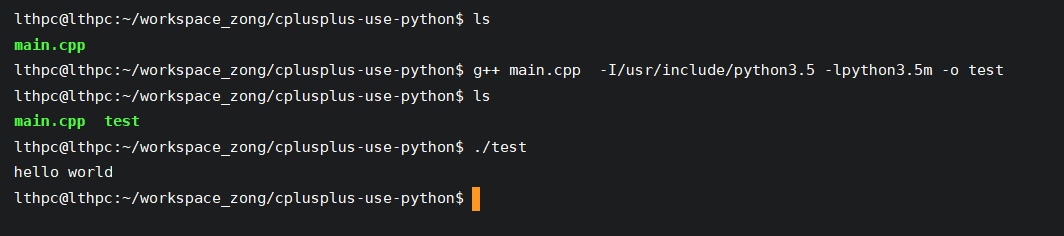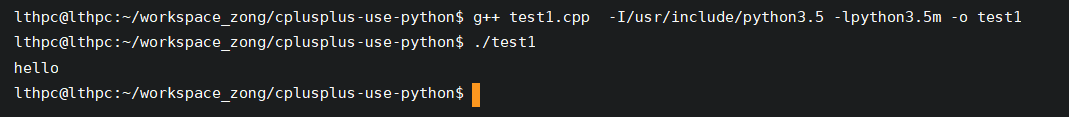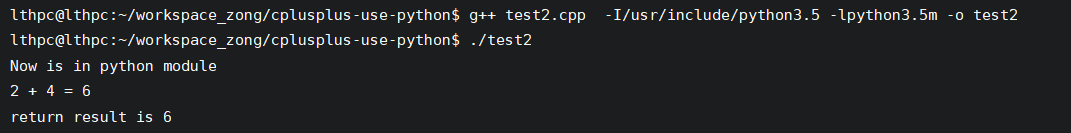# 1 调用流程

c++ 调用 python ，本质上是在 c++ 中启动了一个 python 解释器，由解释器对 python 相关的代码进行执行，执行完毕后释放资源，达到调用目的。

// main.cpp
#include <Python.h>
int main(int argc, char *argv[]) {
// 初始化python解释器.C/C++中调用Python之前必须先初始化解释器
Py_Initialize();

// 执行一个简单的执行python脚本命令
PyRun_SimpleString("print('hello world')\n");

// 撤销Py_Initialize()和随后使用Python/C API函数进行的所有初始化
Py_Finalize();
return 0;
}

g++ main.cpp  -I/usr/include/python3.5 -lpython3.5m -o test# 2 无参函数调用

#include <Python.h>
#include <iostream>

using namespace std;

int main(){
// 1、初始化python接口
Py_Initialize();
if(!Py_IsInitialized()){
cout << "python init fail" << endl;
return 0;
}
// 2、初始化python系统文件路径，保证可以访问到 .py文件
PyRun_SimpleString("import sys");
PyRun_SimpleString("sys.path.append('./script')");

// 3、调用python文件名，不用写后缀
PyObject* pModule = PyImport_ImportModule("sayhello");
if( pModule == NULL ){
return 1;
}
// 4、调用函数
PyObject* pFunc = PyObject_GetAttrString(pModule, "say");
if( !pFunc || !PyCallable_Check(pFunc)){
return 0;
}
//
PyObject_CallObject(pFunc, NULL);
// 5、结束python接口初始化
Py_Finalize();
return 0;
}

python 脚本内容

def say():
print("hello")# 3 有参函数调用

// test2.cpp
#include<Python.h>
#include <iostream>
using namespace std;

int main()
{
Py_Initialize(); //1、初始化python接口

//初始化使用的变量
PyObject* pModule = NULL;
PyObject* pFunc = NULL;
PyObject* pName = NULL;

//2、初始化python系统文件路径，保证可以访问到 .py文件
PyRun_SimpleString("import sys");
PyRun_SimpleString("sys.path.append('./')");

// 在使用这个函数的时候，只需要写文件的名称就可以了。不用写后缀。

//4、调用函数

//5、给python传参数
// 函数调用的参数传递均是以元组的形式打包的,2表示参数个数
PyObject* pArgs = PyTuple_New(2);

// 0：第一个参数，传入 int 类型的值 2
PyTuple_SetItem(pArgs, 0, Py_BuildValue("i", 2));
// 1：第二个参数，传入 int 类型的值 4
PyTuple_SetItem(pArgs, 1, Py_BuildValue("i", 4));

// 6、使用C++的python接口调用该函数
PyObject* pReturn = PyEval_CallObject(pFunc, pArgs);

// 7、接收python计算好的返回值
int nResult;
// i表示转换成int型变量。
// 在这里，最需要注意的是：PyArg_Parse的最后一个参数，必须加上“&”符号
PyArg_Parse(pReturn, "i", &nResult);
cout << "return result is " << nResult << endl;

//8、结束python接口初始化
Py_Finalize();
}

# myadd.py
print("Now is in python module")
print("{} + {} = {}".format(a, b, a+b))
return a + b08-2403-24
06-28
06-118111
09-171万+
12-20552
10-251万+
03-08912
09-18173
10-313万+
08-295978
10-28655
06-102万+
03-271万+